## ISIS 2ISIS Application Documentation

# fx

Printer Friendly View | TOC | Home

Apply generalized arithmetic operations using multiple cube files

 Overview Parameters Example 1 Example 2 Example 3 Example 4 Example 5 Example 6

## Description

Fx allows general arithmetic operations to be performed on an arbitrary number of input cubes. Fx loads whatever input files are specified, applies a user defined equation to those files and writes the results to an output file. The input file can be a single band or multiple band cube file. If the band number is not specified for the multiple band cube file, then the equation is applied to all the bands within the file.

Five files or fewer can be entered for parameters F1 to F5 under the "Input Cube Files" section to specify the input data. A second method to specify data is to include an arbitrary number of files in a file list and enter the list name in the fromlist parameter that is only visible upon selecting the MODE list located under "File I/O Mode." If the user selects "OUPUTONLY" under "File I/O Mode" then an output file is created based on an equation provided by the user. The user will also need to specify the number of lines, samples, and bands of the output file.

The command line parsing has been improved to handle escape sequences and support arrays better. The equation parser is case insensitive, ignores whitespace, and converts all braces to parentheses. Parameter values, when quoted, no longer need the quotes escaped.

The command line syntax changes are as follows (with TCSH/BASH examples):

TCSH/BASH
Before: crop from=\"some file.cub\" to=\"output file.cub\"
Now: crop from="some file.cub" to="output file.cub"

Please note that the dollar sign (\$) still requires a backslash for batchlist variables. Array values, for programs such as spiceinit, will be the same.

TCSH
Before: spiceinit from=input.cub ck='(file1.bc,file2.bc)'
Now: spiceinit from=input.cub ck='(file1.bc,file2.bc)'

BASH
Before: spiceinit from=input.cub ck='(file1.bc,file2.bc)'
Now: spiceinit from=input.cub ck='(file1.bc,file2.bc)'

An escape character "\" has been added to differentiate a first parenthesis from the beginning of an array sequence.

TCSH
Before: fx equation='"(1+2)/2"'
Now: fx equation='\(1+2)/2'

BASH
Before: fx equation='"(1+2)/2"'
Now: fx equation=\(1+2)/2

The equation string entered within a GUI interface may not work if the entire string is copied, pasted, and executed at the command line. If the first character in the equation is a "(" then it must be prefixed by a "\" when executing as a command line, but the remaining parentheses do not need to be prefixed with a backslash.

Example:

This equation string works inside the fx GUI:
fx f1=BIFQF23N004_D218_T069S02_V02_I3.cub to=tt.cub equation=(f1*(f1>.004))

These equation strings fail on the command line:
fx f1=BIFQF23N004_D218_T069S02_V02_I3.cub to=tt.cub equation=(f1*(f1>.004))

fx f1=BIFQF23N004_D218_T069S02_V02_I3.cub to=tt.cub equation="(f1*(f1>.004))"

This equation string works on the command line:
fx f1=BIFQF23N004_D218_T069S02_V02_I3.cub to=tt.cub equation="\(f1*(f1>.004))"

Note: The use of negative numbers within an equation could cause problems in the latest version of ISIS. The "--" or "neg" operators are specifically designed to handle negative numbers within an equation. If an equation includes a negative number, prefix the value with a "--" instead of "-" in the equation.

Example:

equation="f1*(--0.018*sec*(f3*pi/180)^2+f2+4.4234)"

or

equation="f1*(neg(0.018)*sec*(f3*pi/180)^2+f2+4.4234)"

For additional information see Command Line Usage under the User Documentation web pages for ISIS.
Note: Some instructions may be outdated.

The equation parser is case insensitive and all braces, such as "[{[{ }]}]" are converted to parentheses "(((( ))))" first. Whitespace is ignored. Currently, you must explicitly state all multiplication operations (e.g. 2pi will not work, but 2*pi will). The modulus (%), AND, and OR operators are not implemented yet. Functions such as pha, ina, ema, lat, lon, radius, and resolution require a Level1 input file with SPICE information in the image labels. That is, the program spiceinit should be executed on the input files before using these functions. For Level2 images, the backplanes containing the photometric and spatial information must be present. This is normally performed with the program phocube before the image is projected to a map projection.

Requirements and result of operators

The following operators return a "0" or "1" DN value when they are used in the equations:

< , >, <=, >=, ==, !=

The following operators are used to shift pixels left or right, and move the entire image to different sample and line positions:

<<, >>

Example:
Some pixels are set to NULL depending on whether the left or right pixel shift operation is used. For example, the left image below is the input file, and the right image is the result of "equation = f1 << 10", which shows a 10 pixel shift to the left.

Sample 1 line 1 of the left image maps outside the image area of the right image; therefore, sample 11 line 1 of the left image maps to sample 1 line 1 of the right image, and sample 12 line 1 of the left image maps to sample 2 line 1 of the right image. The remaining pixels that do not have an assigned pixel value are set to NULL.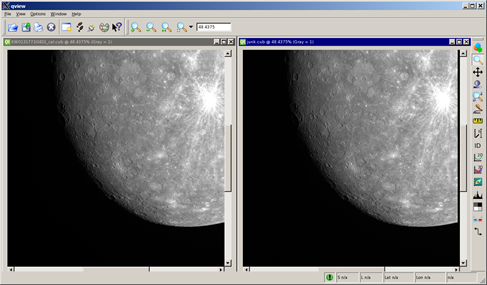The following operators use the input image statistics to apply the equations:

linemin, linemax, cubemin, cubemax, cubeavg, cubestd

The min and max operators are used to compare two pixel values. The pixel values can be obtained from input files or manually entered by the user. The two values are compared against each other and the one that meets the equation criteria is used.

min, max

In order to use the camera operators, images must be processed through spiceinit so that the NAIF camera model information is stored in the image labels. These operators calculate values on a band-by-band basis so that band-dependent images have correctly calculated camera-related values.

pha, phal, phac, ina, inal, inac, ema, emal, emac

The following operators are used to convert between degrees and radians:

All trigonometric functions expect angles in radians, not degrees. However, all camera functions return angles in degrees and therefore should be converted to radians.

The following table shows all currently supported scalars and special tokens:

Scalar Description Example SCALARS F# File operator f3 denotes third file # or #.# or .# Any integer or double 12, 3.14, .007 are all valid band Current band number f1 * band line Current line number line + f1 sample Current sample number sample / line + f1 pi Pi (3.14159...) f1 > (e^pi) e Euler's number (2.71828...) f1 == ln(e)

The following table shows all currently supported operators sorted by precedence (0 = highest precedence). All examples are valid equations that assume one or more files (F1, F2, etc.) are loaded:

PrecedenceLevel Operator Description Example OPERATORS 0 { [ ( ) ] } Parentheses, brackets, or braces f2*(f1+[30-{line/pi}]) 1 -- or neg Negative sign --f1 + f2 or neg(f1) + f2 1 abs Absolute value abs(f2 - f1) 1 min Minimum of two DNs f1 + min(5, f2) + min(f2, f3) 1 max Maximum of two DNs 20 * max(f1,f2) 1 linemin Minimum of DNs on the current line f1 + linemin(f2) 1 linemax Maximum of DNs on the current line f1 + linemax(f2) 1 cubemin Minimum of a cube f1 + cubemin(f2) 1 cubemax Maximum of a cube f1 + cubemax(f2) 1 cubeavg Average of a cube f1 / cubeavg(f1) 1 cubestd Standard deviation of a cube f1 * (abs(f1-cubeavg(f1)) < 2*cubestd(f1)) 1 sin Sine f1 * sin(123/321) 1 cos Cosine cos(.02*50) 1 tan Tangent tan(f1/f2) 1 csc Cosecant 12.3 + csc(f1) 1 sec Secant sin(pi/60) + (sec(f2))^2 1 cot Cotangent line + cot(f1) - 42 1 asin Arcsine 0.006 ^ asin(f1*5) 1 acos Arccosine acos(1/[2*pi]) 1 atan Arctangent atan(f1/e) 1 atan2 Arctangent2 atan2(--10 5.5) 1 sinh Hyperbolic sine 55 + sinh(f2) 1 cosh Hyperbolic cosine cosh(sample^pi) 1 tanh Hyperbolic tangent tanh(f1) 1 log or ln Natural log ln(abs(1/[f2-f1])) 1 log10 Log base 10 99 + log10(f1-160) 1 sqrt Square root sqrt(abs[1000 - f2]) 1 pha Phase angle on the ellipsoid pha(f1) 1 ina Incidence angle on the ellipsoid ina(f1) 1 ema Emission angle on the ellipsoid ema(f1) 1 phal Local phase angle on DTM phal(f1) 1 inal Local incidence angle on DTM inal(f1) 1 emal Local emission angle on DTM emal(f1) 1 phac Phase angle on the ellipsoid at image center phac(f1) 1 inac Incidence angle on the ellipsoid at image center inac(f1) 1 emac Emission angle on the ellipsoid at image center emac(f1) 1 rads Convert degrees to radians f1 / cos(rads(pha(f1))) 1 degs Convert radians to degrees degs(acos(f1)) 1 lat Latitude lat(f1) 1 lon Longitude lon(f1) 1 radius Radius of DTM in meters radius(f1) 1 res Pixel resolution in meters res(f1) 2 ^ Exponent f1 ^ 3 3 * Multiplication 10 * f1 3 / Division f2 / f1 4 << Left shift (Note: pixel shift, not bitwise) f1 << 250 4 >> Right shift (Note: pixel shift, not bitwise) f2 + (f1 >> 500) 5 + Addition 123 + 0.004 + f1 5 - Subtraction 10 - (--f1) 6 > Greater than (Note: result is 0 or 1) f1 > f2 6 < Less than (Note: result is 0 or 1) f1 * (f1 < cubeavg(f1)) 6 <= Less than or equal (Note: result is 0 or 1) f1 <= 0.505 6 >= Greater than or equal (Note: result is 0 or 1) f1 * (f1 >= 101) 6 == Equal to (Note: result is 0 or 1) f1 == (f2/2) 6 != Not equal to (Note: result is 0 or 1) f1 != f2

## History

 Kris Becker 1997-04-24 Original version Sean Crosby 2007-02-14 Converted to Isis 3 Steven Lambright 2007-06-18 Added single line and sample functionality Steven Lambright 2008-04-16 Upgraded to work with new Calculator classes Steven Lambright 2008-12-17 Renamed parameter FILELIST to FROMLIST Steven Lambright 2009-04-17 Updated documentation to reflect the units of the trig functions Steven Lambright 2010-04-08 Min, max capabilities have been expanded to include line and cube min/max Jeff Anderson 2012-02-09 Added cubeavg, cubestd, rads, degs, neg, pha, ina, ema, phac, inac, inal, phal, emal, res, lat, lon, and radius functions Ella Mae Lee 2012-10-22 Improved documentation and added examples, reference Mantis issue #977 and #992 Lynn Weller 2013-02-25 Removed links to applications imbedded in text and replaced with italicized application name. Added application links to the "Related Objects and Documents" section of the documentation. Fixes mantis ticket #1525. Tracie Sucharski 2013-12-11 Fixed bug where the cube attributes on files in the fromlist were ignored. Fixes #1926." Moses Milazzo and Ian Humphrey 2016-10-13 Fixed bug where camera related operators produce incorrect calculations for band-dependent images. The camera operators now make calculations on a band-by-band basis, meaning band-dependent images are now supported with correct calculations. Fixes #1301. Backward Compatibility Issue: The changes made will impact any scripts that use the fx camera operators on band-dependent images, producing different output for each band.

## Parameter Groups

### Files

Name Description
F1Input filename
F2Input filename
F3Input filename
F4Input filename
F5Input filename
TO Output cube filename

### File list

Name Description
FROMLISTInput list filename

### Equation

Name Description
EQUATIONImage processing equation

### File I/O Mode

Name Description
MODEFile I/O options

### Output only

Name Description
LINESNumber of lines
SAMPLESNumber of samples
BANDSNumber of bands

### Files: F1

#### Description

Input ISIS cube filename.

 Type cube input *.cub

### Files: F2

#### Description

Input ISIS cube filename.

 Type cube input None *.cub

### Files: F3

#### Description

Input ISIS cube filename.

 Type cube input None *.cub

### Files: F4

#### Description

Input ISIS cube filename.

 Type cube input None *.cub

### Files: F5

#### Description

Input ISIS cube filename.

 Type cube input None *.cub

### Files: TO

#### Description

This is the output file created based on a user defined equation.

 Type cube output real *.cub

### File list: FROMLIST

#### Description

This file contains a list of all the cube files to be processed. Each file will be assigned F1 to F##(number of images in the input list).

 Type filename input *.txt *.lis

### Equation: EQUATION

#### Description

This equation will be parsed and used to perform the specified calculations.

 Type string

### File I/O Mode: MODE

#### Description

Select one of the following options: CUBES to input cubes directly, LIST to enter a text file containing a list of filenames, or OUTPUTONLY to create output data only.

Type string
Default CUBES
Option List:
Option Brief Description
CUBESSelect input cubes directly Enter up to five input filenames.

#### Exclusions

• FROMLIST
• LINES
• SAMPLES
• BANDS
LISTSpecify a list of input files Input file list name containing all the cube files to be processed.

#### Exclusions

• F1
• F2
• F3
• F4
• F5
• LINES
• SAMPLES
• BANDS
OUTPUTONLYWrite output only Generates output from an equation; no input cubes are needed.

• F1
• F2
• F3
• F4
• F5
• FROMLIST

### Output only: LINES

#### Description

This is the number of lines the output cube will have.

 Type integer 1 1 (inclusive)

### Output only: SAMPLES

#### Description

This is the number of samples the output cube will have.

 Type integer 1 1 (inclusive)

### Output only: BANDS

#### Description

This is the number of bands the output cube will have.

 Type integer 1 1 (inclusive)

### Example 1

#### Description

In this example, two images with the same filename but have two different bands will be added to each other.

#### Command Line

fx f1=../isisTruth.cub+1 f2=../isisTruth.cub+2 to=../result.cub equation=f1+ f2
Add Band 1 and Band 2 using default settings.

#### GUI Screenshot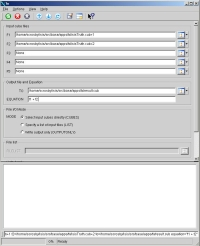fx GUI Example GUI Screenshot of GUI with parameters filled in to perform a calculation on the input image.

#### Input Images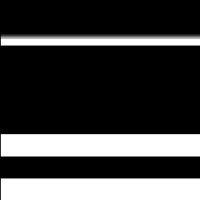Input image Input file band 1 Parameter Name: F1 This is the first input image for the fx example.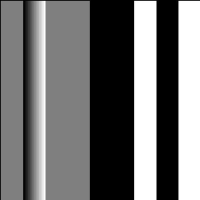Input image Input file band 2 Parameter Name: F2 This is the second input image for the fx example.

#### Output Image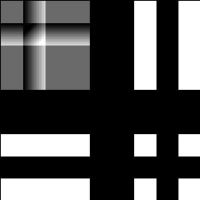Output image Output file Parameter Name: TO This is the 500 by 500 output image containing the results. Parameter Name: EQUATION "f1+ f2"

### Example 2

Create output only

#### Description

In this example, an output file that is 360 samples by 360 lines is created by converting the line number to radians, and then calculating the cosine of the calculated value using the following equation:
The line number is input as degrees and converted to radians first in order to output the correct cosine values.

#### Command Line

fx to=cosine_of_line.cub equation="cos(rads(line))" mode=outputonly lines=360 samples=360
Convert the line number to radians, calculate the cosine of the new value, and output the results to the output filename provided by the user.

#### GUI Screenshot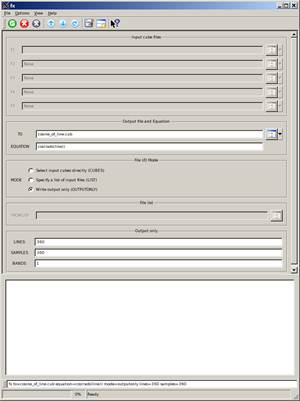fx GUI Example GUI Screenshot of GUI with parameters filled in to perform a calculation to create an output image.

#### Output ImageOutput image Output file Parameter Name: TO This is the output file created based on a user defined equation without specifying input filenames. Parameter Name: EQUATION "cos(rads(line))"

### Example 3

Create output only

#### Description

The example below demonstrates how easily one can get an incorrect product if angles are not converted to radians before calculating the cosine of a line number when the line number represents angles in degrees. In this example, the output file has 360 samples by 360 lines.

#### Command Line

fx to=cosine_of_line_deg.cub equation="cos(line)" mode=outputonly lines=360 samples=360
Calculate the cosine of the line number, and output the results to the output filename provided by the user.

#### GUI Screenshot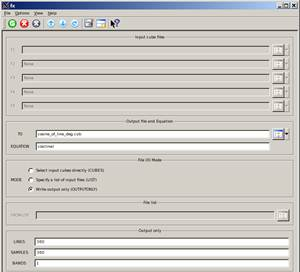fx GUI Example GUI Screenshot of GUI with parameters filled in to perform a calculation to create an output image.

#### Output ImageOutput image Output file Parameter Name: TO This is the output file created based on a user defined equation without specifying input filenames. Parameter Name: EQUATION "cos(line)"

### Example 4

Apply a gamma stretch

#### Description

In this example, a gamma stretch is applied to a CTX image. The image statistics are determined and used as part of the equation to calculate new DN values.

#### Command Line

fx f1=B10_013516_1520_XN_28S285W_eo_reduced.cub to=B10_013516_1520_XN_28S285W_gammast2.cub equation="{[cubemax(f1)-abs(cubemin(f1))]*{[f1-abs(cubemin(f1))]/[cubemax(f1)-abs(cubemin(f1))]}^(1.0/1.8)}"
Apply an equation to perform a gamma stretch to the image utilizing the input image statistics.

#### GUI Screenshot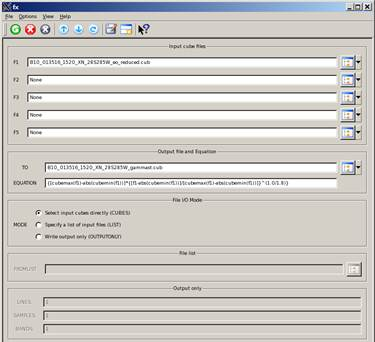fx GUI Example GUI Screenshot of GUI with parameters filled in to perform a calculation on the input image.

#### Input Image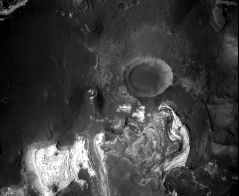Input image Input file Parameter Name: F1 This is the input image for the fx example.

#### Output Image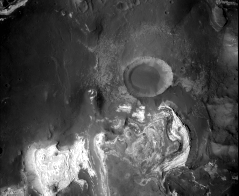Output image Output file Parameter Name: TO This is the output image where the bright and dark tones in the input image are adjusted based on the input image statistics that are incorporated into the equation that is applied to calculate new output values. Parameter Name: EQUATION "{[cubemax(f1)-abs(cubemin(f1))]*{[f1-abs(cubemin(f1))]/[cubemax(f1)-abs(cubemin(f1))]}^(1.0/1.8)}"

### Example 5

#### Description

In this example, all input DN values greater than 0.11 is set to "1.0" and all other pixels set to "0.0".

#### Command Line

Apply the equation to create a mask template file consisting of 0.0 and 1.0 DN values.

#### GUI Screenshot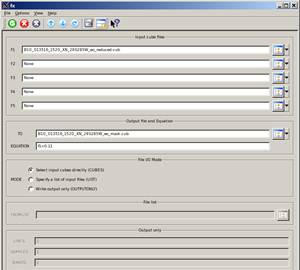fx GUI Example GUI Screenshot of GUI with parameters filled in to perform a calculation on the input image.

#### Input ImageInput image Input file Parameter Name: F1 This is the input image for the fx example.

#### Output Image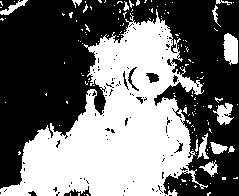Output image Output file Parameter Name: TO This is an image consisting of "0" and "1" DN values based on the equation provided. Parameter Name: EQUATION "f1>0.11"

### Example 6

Extract desired data

#### Description

In this example, all DN values less than 0.11 will be set to "0.0" and all other DN values will retain the original input value.

#### Command Line

fx f1=B10_013516_1520_XN_28S285W_eo_reduced.cub to=B10_013516_1520_XN_28S285W_extract.cub equation="f1*(f1>0.11)"
Apply the equation to retain all DN values greater than 0.11, and set all other values to 0.0.

#### GUI Screenshot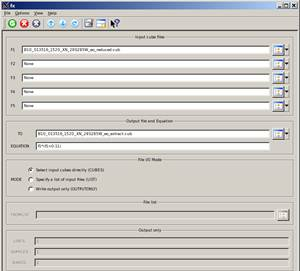fx GUI Example GUI Screenshot of GUI with parameters filled in to perform a calculation on the input image.

#### Input ImageInput image Input file Parameter Name: F1 This is the input image for the fx example.

#### Output Image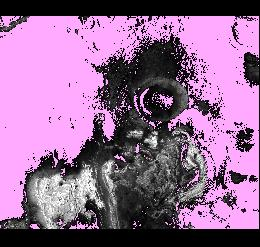Output image Output file Parameter Name: TO The pink areas in this image were set to "0.0" based on the equation entered. All other values retained the original values because the input value was multiplied by 1.0 when the calculations were performed. Parameter Name: EQUATION "f1*(f1>0.11)"